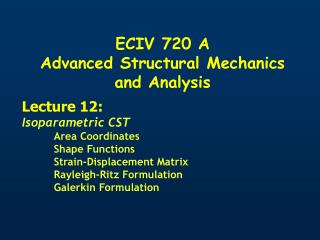DownloadDownload PresentationECIV 720 A Advanced Structural Mechanics and Analysis

# ECIV 720 A Advanced Structural Mechanics and Analysis

Download Presentation## ECIV 720 A Advanced Structural Mechanics and Analysis

- - - - - - - - - - - - - - - - - - - - - - - - - - - E N D - - - - - - - - - - - - - - - - - - - - - - - - - - -
##### Presentation Transcript

1. ECIV 720 A Advanced Structural Mechanics and Analysis Lecture 12: Isoparametric CST Area Coordinates Shape Functions Strain-Displacement Matrix Rayleigh-Ritz Formulation Galerkin Formulation

2. FEM Solution: Area Triangulation Area is Discretized into Triangular Shapes

3. FEM Solution: Area Triangulation One Source of Approximation

4. FEM Solution: Nodes and Elements Points where corners of triangles meet Define NODES

5. Non-Acceptable Triangulation … Nodes should be defined on corners of ALL adjacent triangles

6. FEM Solution: Nodes and Elements vi ui Y X Each node translates in X and Y

7. FEM Solution: Objective v q6 u q5 3 (x3,y3) q4 q1 q3 q2 2 (x2,y2) 1 (x1,y1) • Use Finite Elements to Compute Approximate Solution At Nodes • Interpolate u and v at any point from Nodal values q1,q2,…q6

8. Intrinsic Coordinate System 3 (x3,y3) h x 2 (x2,y2) 1 (x1,y1) h x Map Element Define Transformation 2 (0,1) 3 (0,0) 1 (1,0) Parent

9. Area Coordinates 2 Y A1 P X 3 1 Location of P can be defined uniquely Area Coordinates

10. Area Coordinates and Shape Functions 3 (x3,y3) x 2 (x2,y2) h 1 (x1,y1) h 2 (0,1) x 3 (0,0) 1 (1,0) Area Coordinates are linear functions of x and h Are equal to 1 at nodes they correspond to Are equal to 0 at all other nodes Natural Choice for Shape Functions

11. Shape Functions 3 (x3,y3) Y X 2 (x2,y2) x 1 (x1,y1) h

12. Geometry from Nodal Values

13. Intrinsic Coordinate System 3 (x3,y3) Map Element Transformation h x 2 (x2,y2) 2 (0,1) 1 (x1,y1) h x 3 (0,0) 1 (1,0) Parent

14. Displacement Field from Nodal Values 3 q6 v u q5 1 2 x h q4 q2 q3 q1

15. Strain Tensor from Nodal Values of Displacements Need Derivatives Strain Tensor u and v functions of x and h

16. Jacobian of Transformation J Jacobian of Transformation J

17. Jacobian of Transformation – Physical Significance

18. Jacobian of Transformation – Physical Significance 3 (x3,y3) r1 r2 x 2 (x2,y2) 1 (x1,y1) h

19. Jacobian of Transformation – Physical Significance k 3 (x3,y3) r1 r2 x 2 (x2,y2) 1 (x1,y1) h Compare to Jacobian

20. Jacobian of Transformation Solve Solve

21. Strain Tensor from Nodal Values of Displacements

22. Strain Tensor from Nodal Values of Displacements

23. Strain Tensor from Nodal Values of Displacements q B e= B q Looks Familiar?

24. Strain-Displacement Matrix 3 (x3,y3) 2 (x2,y2) x 1 (x1,y1) h Is constant within each element - CST

25. Stresses e= B q

26. Element Stiffness Matrix ke 3 (x3,y3) 2 (x2,y2) x 1 (x1,y1) h e= B q s= DB qe ke

27. Formulation of Stiffness Equations y T (force/area) x z Tt(force/length) y P P x t Assume Plane Stress

28. Total Potential Approach Tt(force/length) P Total Potential

29. Total Potential Approach Tt(force/length) P

30. Total Potential Approach

31. Total Potential Approach Work Potential of Body Forces

32. WP of Body Forces 2 Element e fx u fy 3 v 1

33. WP of Body Forces 3 q6 v u q5 1 2 x h q4 q2 q3 q1

34. WP of Body Forces 3 q6 v u q5 1 2 x h q4 q2 q3 q1

35. WP of Body Forces Nodal Equivalent Body Force Vector

36. Total Potential Approach Work Potential of Tractions

37. WP of Traction Tt(force/length) 2 3 1 Distributed Load acting on EDGE of element

38. WP of Traction Components Normal Pressure Tx1,Ty1 p1, p2 Known Distribution Known Distribution Tx2,Ty2

39. WP of Traction Directional cos Ty2 Tx2 Ty1 Tx1 Normal Pressure p1, p2 Known Distribution Components

40. WP of Traction 2 v Ty u 3 Tx 1

41. WP of Traction Ty1 Ty2 Tx1 Tx2 2 3 1

42. WP of Traction Nodal Equivalent Traction Vector

43. Total Potential Approach Work Potential of Concentrated Loads

44. WP of Concentrated Loads P Indicates that at location of point loads a node must be defined

45. In Summary

46. After Superposition Minimizing wrt Q

47. Galerkin Approach Tt(force/length) P Galerkin

48. Galerkin Approach Tt(force/length) P

49. Galerkin Approach 3 y6 fy fx y5 1 2 x h y4 y2 y3 y1 Introduce Virtual Displacement Field f

50. Galerkin Approach Virtual Strain Energy of element e